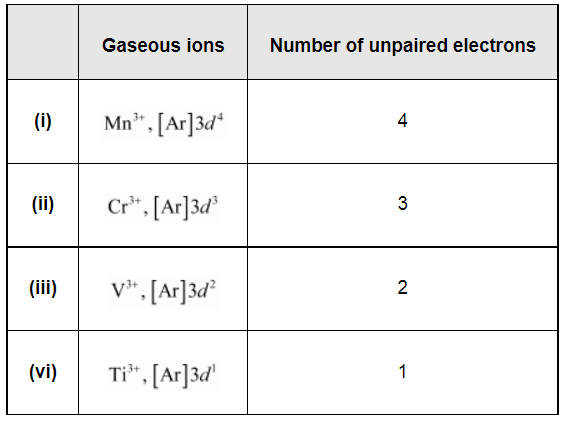# Calculate the number of unpaired electrons in the following gaseous ions:Question:

Calculate the number of unpaired electrons in the following gaseous ions: Mn3+, Cr3+, V3+ and Ti3+. Which one of these is the most stable in aqueous solution?

Solution:$\mathrm{Cr}^{3+}$ is the most stable in aqueous solutions owing to a $t_{2 \mathrm{~g}}^{3}$ configuration.